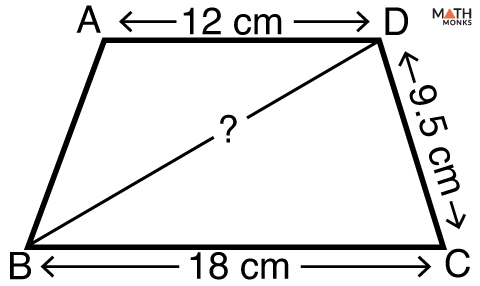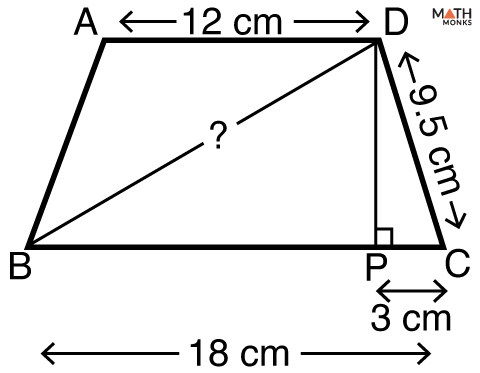# Diagonals of a Trapezoid

The diagonals of a trapezoid are the straight line segments linking its opposite vertices or corners.

Are the diagonals of any trapezoid are congruent?

NO. The diagonals of any trapezoid is not congruent. Let us learn when the diagonals of a trapezoid are congruent.

## Diagonals of an Isosceles Trapezoid

The diagonals of an isosceles trapezoid are congruent. This is a special case of a trapezoid when its 2 legs are equal in length. So the diagonals are also congruent.

Do the diagonals of a trapezoid bisect each other?

NO. The diagonals a trapezoid do not bisect each other.

Let us solve an example to understand the concept better.Find the length of the diagonal of an isosceles trapezoid given.Solution:

As we know,
Long base (a) = 18 cm, short base (b) = 12 cm
Now,
We draw in a perpendicular to BC at P.
Now,
a – b
= 18 – 12
= 6 cm
∴ PC = 6/2 = 3 cm
Applying Pythagorean’s Theorem,
In ΔDPC,  DP2 + PC2 = DC2
DP2 = √(DC2 – PC2)
= √(9.52 – 32)
= 9.01 cm
Now in ΔBPD,  BD2 = BP2 + DP2
BP = BC – PC
= 18 – 3
= 15 cm
BD = √(BP2 + DP2)
= √(152 + 9.012)
= √(225 + 81.2)
≈ 17.50 cm